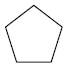Name:    2nd Grade Math Basic Test 3

Multiple Choice
Identify the choice that best completes the statement or answers the question.

1.

6a. 8 R4 d. 8 R3 b. 9 R2 e. 7 R10 c. 7 R4

2.

Round 68 to the nearest tens place.
 a. 78 d. 80 b. 70 e. 100 c. 60

3.

243
x15
 a. 1,458 d. 3,635 b. 5,645 e. 3,545 c. 3,645

4.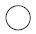Same shape and size as:
 a.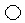d.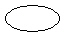b.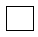e.c.5.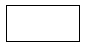Same shape as:
 a.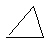d.b.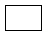e.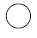c.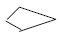6.

Geometry – Shapes and Figures – RIT 151 – 160
Which of these is a triangle?
 a.c.b.d.7.

Geometry – Shapes and Figures – RIT 161 – 170
Which shape below is a cone?
 a.d.b.e.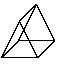c.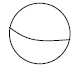8.

Which figure is made of all square sides?
 a.c.b.9.Number of sides?
 a. 2 d. 1 b. 4 e. 5 c. 3

10.

Which is a closed figure?
 a.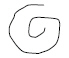d.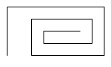b.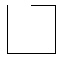e.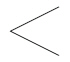c.11.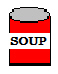A can has:
 a. two circles d. a rectangle side b. square sides e. two square sides c. two triangles

12.

Geometry – Congruency and Similarity – RIT 161 – 170
Which has congruent shapes?
 a.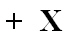d.b.e.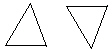c.13.

Geometry – Shapes and Figures – RIT 171 – 180
Which is a cylinder?
 a. shoebox d. soup can b. baseball e. book c. dice

14.

Geometry – Symmetry and Transformations – RIT 171 – 180
Which of these shows a flip?
 a.d.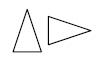b.e.c.15.

Geometry – Congruency and Similarity – RIT 171 – 180
Which shape is congruent to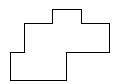a.d.b.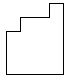e.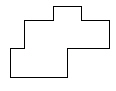c.